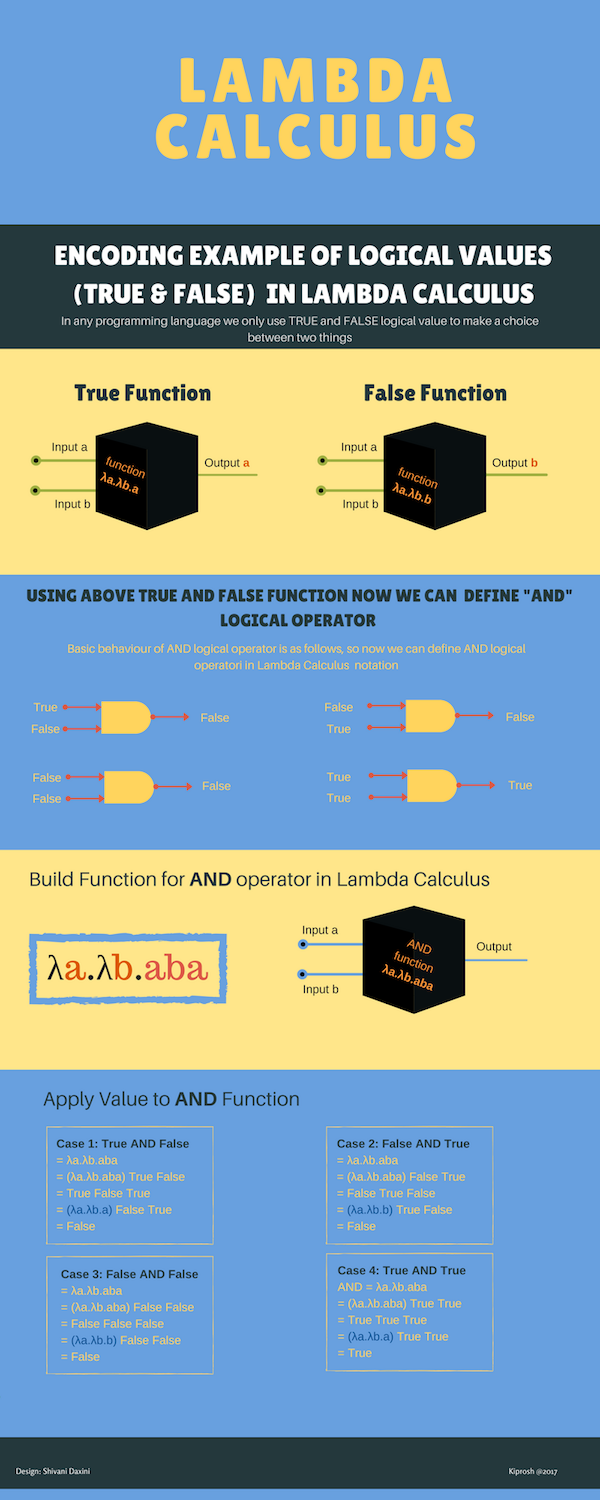I have been writing code in Imperative programming languages for many years. Writing code in functional programming is a big shift and change in thinking. As I recently started with Elixir and when I was reading about Functional Programming, I keep reading mention of Lambda Calculus every time. That ignited my curiosity to brush up my computer science knowledge.

As Functional programming languages implement Lambda Calculus(λ-calculus). If we understand how Lambda Calculus notation works from Computer Science perspective it will be easier to understand Functional programming. As nowadays most of the languages have implementation of lambda calculus may it be a functional language or a Imperative programming language

So here is background about Lambda Calculus. It was invented by Alonzo Church in 1930s to understand the notion of Functions from Computer science point of view.

### Function

In Lambda Calculus function is nothing but a Black Box. You cannot see what is happening inside the function and how data is processed. You can just give an input and observe the returned output.

### Pure Function

Lambda Calculus also explains concept of Pure Function easily. As mentioned above in the definition of function, we can give input, observe output and we cannot save or store state internally. That makes a function Pure Function. So if you call a function 100 times with same input value, it will always return you the same output. Previous function call will not have any impact on the next call of the function result. Pure Functions only allow substitution model.

### Three rules of Lambda Calculus

``````• Variables
• Build function/Abstraction
• Apply Function/Application
``````

Lambda calculus has only these three things and other than it does not have anything like data types, looping, control structure or recursion etc.

As per Lambda Calculus , you just need these three rules and you can encode anything, yes you heard right you can encode any computation. The solution which we write in Lambda calculus might not always a best or performant one, but it can encode anything.

So this is the computational model on which Functional programming is based. Everything which we mentioned above applicable to Functional programming.

Nowadays, you can write functional program in any language, it is not necessary you should only use a functional language. You can even write functional program in C#, java etc. as all these language now use lambda calculus

Now I will share with you how to encode in Lambda Calculus and how to use it. Lets say we want to implement AND logical operator in Lambda Calculus.

As you know when we write any logical code we decide whether we choose one thing or the another. So for this we will build two Lambda calculus function definitions, to get True as output and another to get False .

Refer the infographic for the complete explanation of the Logical functions True & False, on top of it build AND logical operator function and apply inputs to the build function.